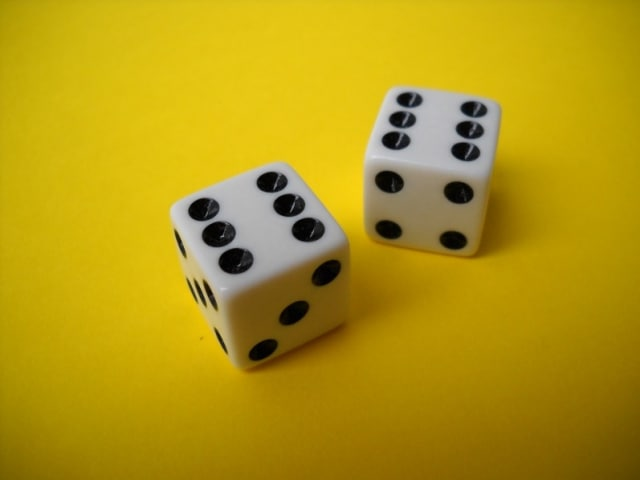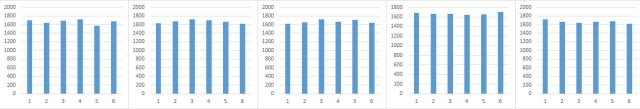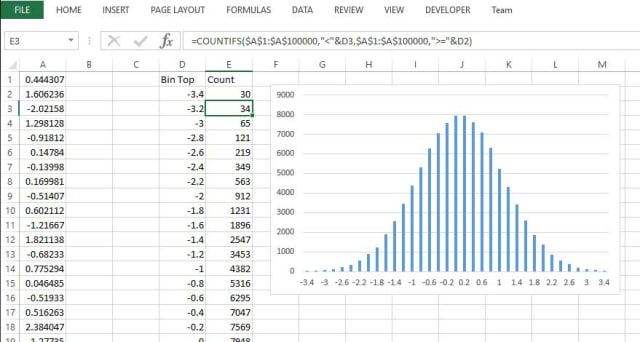Introduction to Monte Carlo Simulation
Dr Jody Muelaner posted on January 15, 2019 |
What a Monte Carlo simulation is and how to perform one in Microsoft Excel.In my recent article, why simulation is the future of uncertainty evaluation, I explained why traditional analytical methods can sometimes be inaccurate. It is for this reason that the latest standards recommend that simulations are used to verify models. In this article I will explain exactly what a Monte Carlo simulation is and how you can perform one. I will also explain how you can estimate the size of errors in the simulation.

Monte Carlo simulation involves using random number generators to simulate random effects. Simulating an event many times allows us to measure the variation just as we would if we took many samples of a real event. In everyday life, the most common random number generators are dice, for this reason dice are often used to represent Monte Carlo simulation. In real simulations, random number generators are software functions within a computer program. These digital random number generators can produce any value from a given probability distribution and millions of values can be created in under a second. This can enable many complex effects to be simulated far more quickly than actual experiments could be performed.

### A simple example

Let’s start with a very simple example. Let us suppose we want to know what happens when we roll two dice, and in particular, what is the probability of getting two sixes. In this simple example it is not difficult to apply the rules of probability to determine the result. You probably remember that the probability of one event occurring and then being followed by a second event is given by the probabilities of each event multiplied together. Assuming the dice are fair, there is a one in six chance of getting a six on each roll. The probability of getting two sixes is therefore 1/6 x 1/6 = 1/36.Figure 1: If we roll the dice many times we can find the probability of getting two sixes, this is the essence of a Monte Carlo Simulation

We could of course also find this result experimentally by simply rolling the dice many times. Since there is only a one in 36 chance of scoring two sixes this would take quite a long time. If we rolled the dice 50 times we might see the event of two sixes once or twice but this would not be enough observations to give an accurate estimate of the probability. For this, we would need to observe the event of getting two sixes many times, so we would need to roll the dice several hundred or ideally thousands of times.

Using Monte Carlo simulation we can apply essentially the same approach as carrying out an experiment. To do this we first need to simulate the role of the dice. This is done using a random number generator which is a computer algorithm which produces a random number. Technically, these are generally pseudorandom number generators (PRNG) since eventually the generated numbers will repeat in a pattern. Seed numbers will determine the pattern of numbers produced and may be provided from sources such as user input or the computer clock. To use the Monte Carlo approach, we don’t need to fully understand how the PRNG works but we should be aware that some functions will produce numbers that repeat quite quickly. Such functions are only suitable for applications like games. High quality algorithms may be specified as suitable for cryptography or statistical applications.

### Simulating a single dice roll

In this example, we will use the RAND function in Microsoft Excel. This is of moderate quality in older versions of Excel, being suitable for sample sizes of less than 1 million. Since Excel 2003 a very high quality algorithm is used which has been show by NIST to be suitable for samples of up to 10^13. The RAND function has no arguments and returns a value between zero and one, to 15 decimal places, with a uniform probability distribution. To simulate a dice we need to return an integer(whole number) between one and six. This can be achieved using the ROUND function to give integer values as shown below:

=ROUND(0.5+6*RAND(),0)

Alternatively, Excel has a built-in function, RANDBETWEEN, which will achieve the same result:

=RANDBETWEEN(1,6)

Either of these functions will return an integer between one and six with a uniform probability, just like a six sided dice. We can validate that these are performing correctly by first simulating a single dice roll many times. This will be our first, very simple, Monte Carlo simulation. First, insert one of the equations, given above, into cell A1 of a new worksheet. Now, select cell A1 press Ctrl + C to copy its contents. Next, click on the name box in the top left of the workbook, type the range A2:A10000 and hit enter to select this range. Finally, pressCtrl + V to copy this equation into the whole range.Figure 2: using the name box to select a large range of cells to copy the dice roll simulation formula

At this stage, column A should contain 10,000 simulated dice rolls. We can create a table which counts how many times each score occurred using the COUNTIF function. Finally, we can create a bar graph which provides a neat graphical representation of whether the dice is fair. A fair dice will produce an approximately equal count for each score.Figure 3: using COUNTIF to count how many times each score occurred and then charging these results

Congratulations, you have now created a Monte Carlo simulation. If you press F9 all the values will be updated running an entirely new simulation. Try pressing F9 a few times and watch the values change as well as the heights of the bars in the charts. Each time the chart refreshes a new simulation has been performed, including 10,000 simulated rolls of the dice. A little quicker than performing the actual experiment!

To get a feel for how this simulation works, and how many samples are required to obtain an accurate result, try adjusting the range of values which are counted. This is equivalent to changing the sample size for experimental data. Some examples are shown in the figure below. Figure 4 shows the results of 5 different simulations, each with 100 dice rolls, this is not enough to give an accurate indication of the probability of each score. It takes about 10,000 rolls to give a reasonable indication of the accuracy. Performing simulations like this can really bring home how small sample sizes in real experiments can result in false conclusions.Figure 4: Scores when rolling a dice 100 timesFigure 5: Scores when rolling a dice 1,000 timesFigure 6: Scores when rolling a dice 10,000 times

### Simulating two dice

Now that we have a way of stimulating a dice roll we can extend this to simulate rolling two dice. We can simply copy column A to simulate the second dice in column B. We can then sum the score from each dice in column C. Each row now gives a simulated roll two dice with the resulting score.Figure 7: simulation of two dice

### Simulating normally distributed values

Typically, when we wish to simulate some event which involves random variations, the variation does not take integer values like a dice. More usually, a variable value will be represented by its mean and standard deviation, and values will vary according to some probability distribution, most commonly the normal, or Gaussian, distribution. Such values can be simulated using a standard uniform random number generator. The most common method is known as the Box–Muller transform, this is a simple equation which takes uniform random numbers as input variables. It can be implemented in Excel using the following formula:

=SQRT(-2*LN(RAND()))*COS(2*PI()*RAND())

To try it out, copy this equation into 100,000 values in column A. Then create a histogram of these values by counting the number of values between an upper and lower limit as shown below.Figure 8: simulating values from the normal distribution

You can see that the distribution has a mean of zero and standard deviation of one, it is the standard normal distribution. Values from any normal distribution can be created by simply multiplying by the standard deviation and adding the mean.

### A more realistic simulation

For the final example in this article we will simulate something a bit more realistic. I’ve previously used the example of measuring the height of a building using a tape measure to measure horizontal distance to a viewing point and a clinometer to measure the angle. In this case, there is uncertainty in both the distance and angle measurements, and we wish to know the uncertainty in the height measurement. I previously showed that sensitivity coefficients are required when using the analytical approach. If we simulate this measurement we only need to know the equation used to calculate the height and the uncertainties in the input variables. The height of the building, H, it is given by:

H = h1 + L tan (a)

Where h1 is the height of the clinometer, L is the horizontal distance along the ground and a is the angle. These inputs have been measured so that h1 is 1.6 m with a standard uncertainty of 4.375 mm, L is 10 m with a standard uncertainty of 25.25 mm and a is 58 degrees with a standard uncertainty of 1 degree. We can simulate each of these values by multiplying the Box–Muller transform by the standard uncertainty and adding the measured value. When doing this it is important to use consistent units. The simulated height is then given using the above equation with the simulated values as inputs. Since the angle is specified in degrees and Excel’s trigonometric functions use radians, the values must be converted.Figure 9: Simulation of a measurement with uncertainty

The final step in this simulation is to find the mean and standard deviation for the values of H in column D. This standard deviation is the standard uncertainty for the measurement of the building’s height. You might also like to create a histogram, as I have done, to check that H follows a normal distribution. Other tests might include calculating the skewness and ketosis, or performing more advanced goodness of fit calculations for the normal distribution. Just because all the input quantities are normally distributed we cannot assume that the output distribution will also be normally distributed.

### Conclusion

In this article, I’ve introduced the concept of Monte Carlo simulation. Using simple examples of dice rolls should make the basic idea easy to understand. Of course we don’t want to simulate dice all that often. Simulating random variables which follow standard distributions such as the normal distribution or the uniform distribution is much more useful. I’ve also given you some basic tools to simulate these types of variable. I also showed that simply repeating the same simulation a number of times is likely to give a slightly different result. The extent of these differences gives an indication of how accurate the simulation is. Generally quite large simulations are required to give stable results. Monte Carlo simulation is an extremely useful and versatile technique for understanding variation in manufacturing processes and uncertainty in measurements. There is a lot more that can be done with Monte Carlo simulation, something I will explore over the next few months.

 Recommended For You